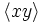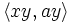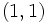# Subgroup structure of central product of D8 and Z4

This article gives specific information, namely, subgroup structure, about a particular group, namely: central product of D8 and Z4.
View subgroup structure of particular groups | View other specific information about central product of D8 and Z4

The central product of the dihedral group of order eight and cyclic group of order four is a central product of these groups, over a common central subgroup of order two.

It is given by the presentation:$G := \langle a,x,y \mid a^4 = x^2 = e, a^2 = y^2, xax = a^{-1}, ay = ya, xy = yx \rangle$.

Here,$\langle a,x \rangle$ is the dihedral group of order eight and$\langle y \rangle$ is the cyclic group of order four.

Here is a quick description of all the subgroups of this group:

1. The trivial subgroup. Isomorphic to trivial group. (1)
2. The subgroup$\langle a^2 \rangle$. This is the unique normal subgroup of order two, and is contained in the center. Isomorphic to cyclic group:Z2. (1)
3. The subgroups$\langle x \rangle$,$\langle ax \rangle$,$\langle a^2x \rangle$,$\langle a^3x \rangle$. These come in two conjugacy classes of 2-subnormal subgroups, one comprising$\langle x \rangle$ and$\langle a^2x \rangle$ and the other comprising$\langle ax \rangle$ and$\langle a^3x \rangle$. However, they are all automorphic subgroups. Isomorphic to cyclic group:Z2. (4)
4. The subgroups$\langle ay \rangle$ and$\langle a^3y \rangle$. These form a single conjugacy class of 2-subnormal subgroups. Isomorphic to cyclic group:Z2. (2)
5. The subgroup$\langle y \rangle$ of order four. This is the center. Isomorphic to cyclic group:Z4. (1)
6. The subgroups$\langle a \rangle$,$\langle xy \rangle$ and$\langle axy \rangle$. These are normal subgroups but are automorphic subgroups: they are related by outer automorphisms. Isomorphic to cyclic group:Z4. (3)
7. The subgroup$\langle a^2, x \rangle$,$\langle a^2, ax \rangle$ and$\langle a^2, ay \rangle$. These are all normal subgroups but are related by outer automorphisms. Isomorphic to Klein four-group. (3)
8. The subgroup$\langle a, xy \rangle$. This is an isomorph-free subgroup of order eight, containing the three non-characteristic cyclic subgroups of order four. Isomorphic to quaternion group. (3)
9. The subgroups$\langle a,y \rangle$,$\langle x, y \rangle$ and$\langle ax, y \rangle$. These are all normal and related by outer automorphisms. Isomorphic to direct product of Z4 and Z2. (3)
10. The subgroups$\langle a,x \rangle$,$\langle xy, ay \rangle$ and$\langle axy, ay \rangle$. These are all normal and are related by outer automorphisms. Isomorphic to dihedral group:D8. (3)
11. The whole group. Isomorphic to central product of D8 and Z4. (1)

## Tables for quick information

### Table classifying isomorphism types of subgroups

Group name GAP ID Occurrences as subgroup Conjugacy classes of occurrence as subgroup Occurrences as normal subgroup Occurrences as characteristic subgroup
Trivial group$(1,1)$ 1 1 1 1
Cyclic group:Z2$(2,1)$ 7 4 1 1
Cyclic group:Z4$(4,1)$ 4 4 4 1
Klein four-group$(4,2)$ 3 3 3 0
Direct product of Z4 and Z2$(8,2)$ 3 3 3 0
Dihedral group:D8$(8,3)$ 3 3 3 0
Quaternion group$(8,4)$ 1 1 1 1
Central product of D8 and Z4$(16,13)$ 1 1 1 1
Total -- 23 20 17 5

### Table listing number of subgroups by order

Group order Occurrences as subgroup Conjugacy classes of occurrence as subgroup Occurrences as normal subgroup Occurrences as characteristic subgroup$1$ 1 1 1 1$2$ 7 4 1 1$4$ 7 7 7 1$8$ 7 7 7 1$16$ 1 1 1 1
Total 23 20 17 5